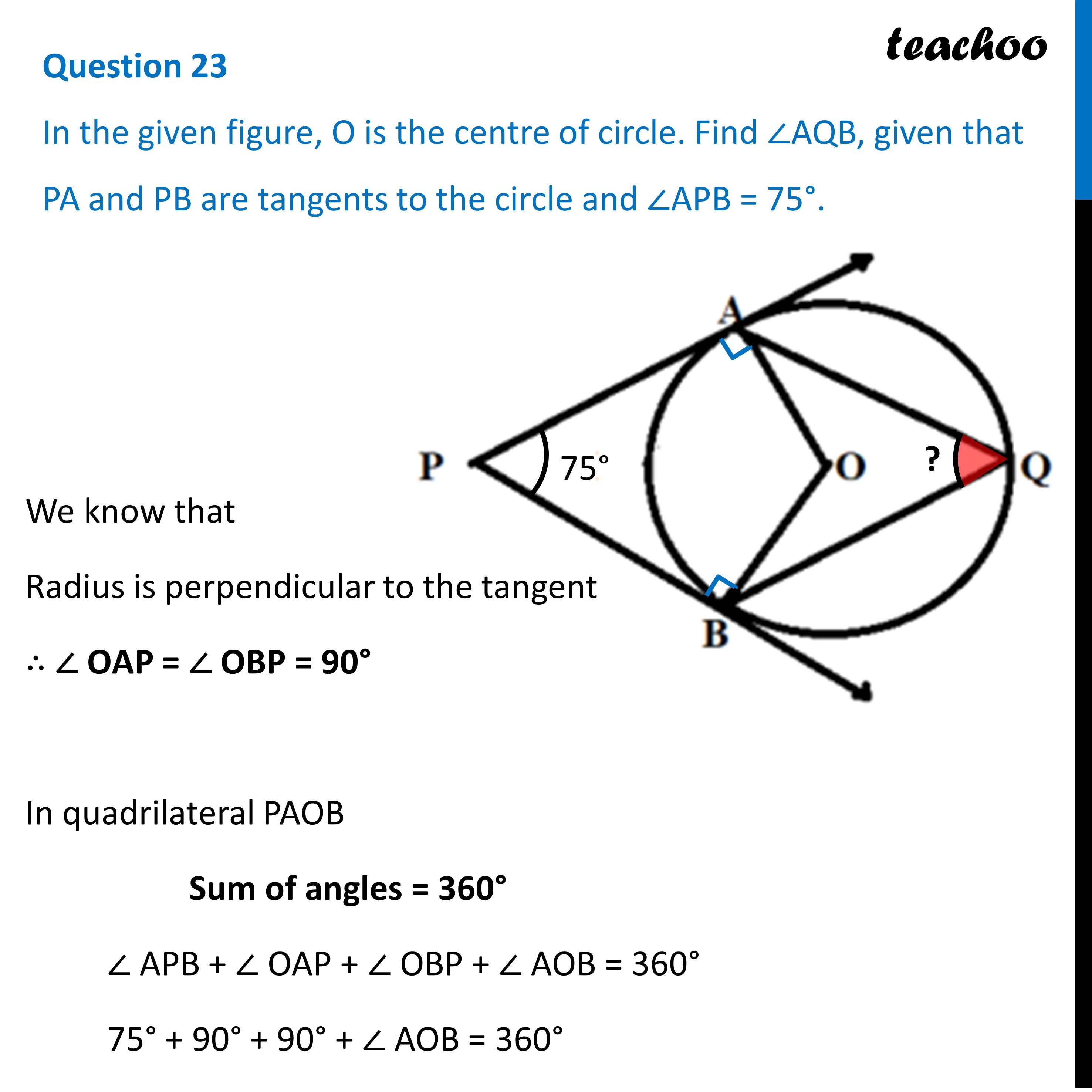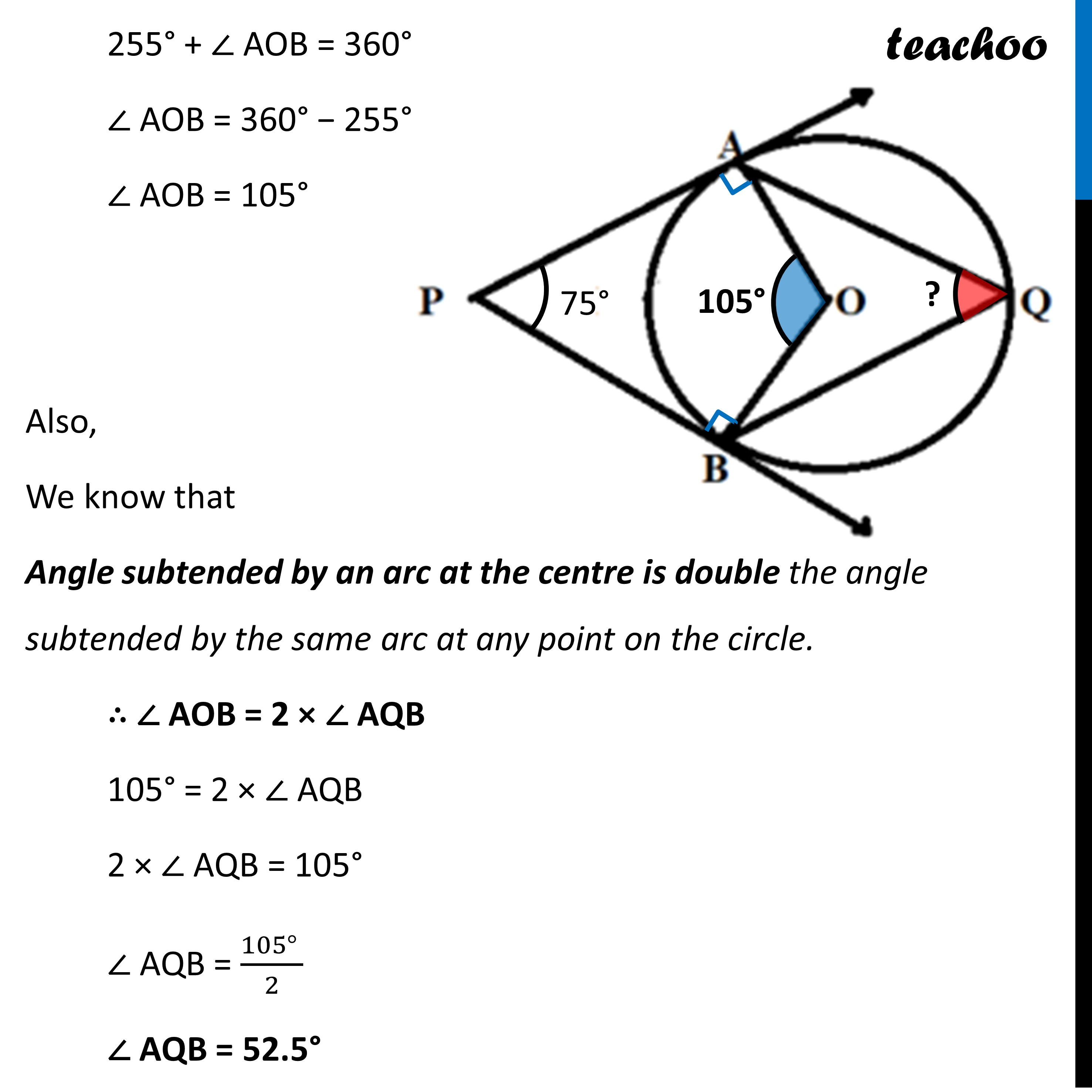CBSE Class 10 Sample Paper for 2023 Boards - Maths Standard

Class 10
Solutions of Sample Papers for Class 10 Boards

## In the given figure, O is the centre of circle. Find ∠AQB, given that PA and PB are tangents to the circle and ∠APB = 75°.Learn in your speed, with individual attention - Teachoo Maths 1-on-1 Class

### Transcript

Question 23 In the given figure, O is the centre of circle. Find ∠AQB, given that PA and PB are tangents to the circle and ∠APB = 75°. We know that Radius is perpendicular to the tangent ∴ ∠ OAP = ∠ OBP = 90° In quadrilateral PAOB Sum of angles = 360° ∠ APB + ∠ OAP + ∠ OBP + ∠ AOB = 360° 75° + 90° + 90° + ∠ AOB = 360° 255° + ∠ AOB = 360° ∠ AOB = 360° − 255° ∠ AOB = 105° Also, We know that Angle subtended by an arc at the centre is double the angle subtended by the same arc at any point on the circle. ∴ ∠ AOB = 2 × ∠ AQB 105° = 2 × ∠ AQB 2 × ∠ AQB = 105° ∠ AQB = (105° )/2 ∠ AQB = 52.5°